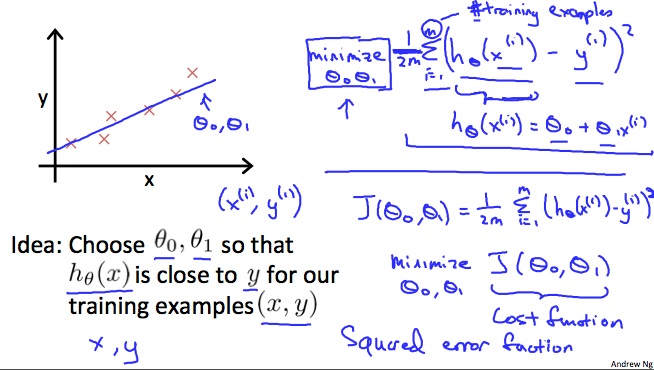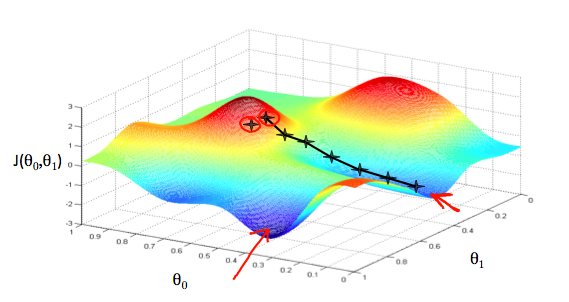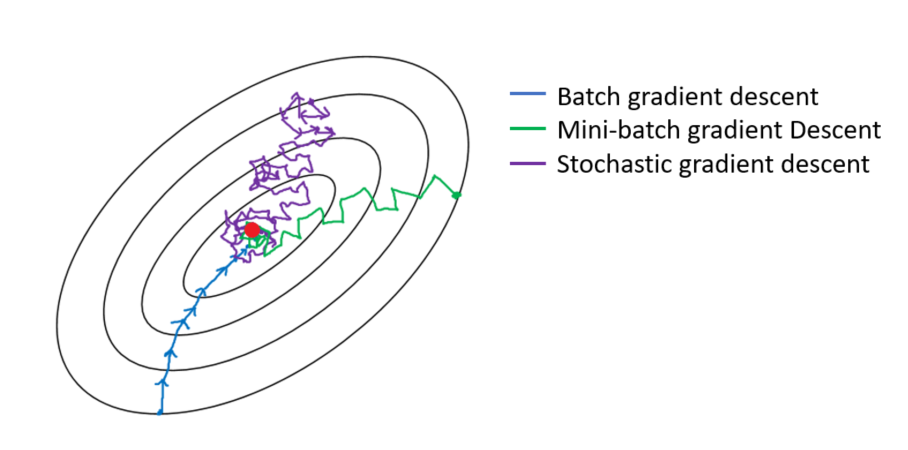# Gradient Descent Mathematics with Python Code

Gradient descent is a first-order iterative optimization algorithm for finding a local minimum of a differentiable function. To find a local minimum of a function using gradient descent, we take steps proportional to the negative of the gradient of the function at the current point. ( Almost every machine learning algorithm has an optimization algorithm at its core.)

In machine learning, we use gradient descent to update the parameters of our model.

Let’s suppose we have a hypothesis( a candidate model that approximates a target function for mapping inputs to outputs) function and we have a way of measuring how well it fits into the data. Now we need to estimate the parameters in the hypothesis function. That's where gradient descent comes in.

Imagine that we graph our hypothesis function based on its fields θ0 and θ1​ (actually we are graphing the cost function as a function of the parameter estimates). We are not graphing x and y itself, but the parameter range of our hypothesis function and the cost resulting from selecting a particular set of parameters.

We put θ0​ on the x-axis and θ1​ on the y-axis, with the cost function on the vertical z-axis. The points on our graph will be the result of the cost function using our hypothesis with those specific theta parameters.

## What is the cost function?

We can measure the accuracy of our hypothesis function by using a cost function. This takes an average difference (actually a fancier version of an average) of all the results of the hypothesis with inputs from x's and the actual output y's.

J(θ0​,θ1​)=2m1​i=1∑m​(y^​i​−yi​)2=2m1​i=1∑m​(​(xi​)−yi​)

This figure explains what a cost function does.The graph below depicts such a setup of Gradient Descent.We will know that we have succeeded when our cost function is at the very bottom of the pits in our graph, i.e. when its value is the minimum. The red arrows show the minimum points in the graph.

The way we do this is by taking the derivative (the tangential line to a function) of our cost function. The slope of the tangent is the derivative at that point and it will give us a direction to move towards. We make steps down the cost function in the direction with the steepest descent. The size of each step is determined by the parameter α, which is called the learning rate.

The gradient descent algorithm is:

repeat until convergence:

θ j := θ j -  α(a/a θj) J(θ0​,θ1 )

where j=0,1 represents the feature index number.

At each iteration j, one should simultaneously update the parameters θ1​,θ2​,...,θn​. Updating a specific parameter prior to calculating another one on the j^{(th) iteration would yield to the wrong implementation.

## Types of Gradient Descent

1. ### Batch Gradient Descent

It is when we sum up overall examples on each iteration when performing the updates to the parameters. Therefore, for each update, we have to sum over all examples,

Algo-

``````for i in range(num_epochs):
params = params — learning_rate * grad``````
1. ### Mini-batch Gradient Descent

Instead of going over all examples, Mini-batch Gradient Descent sums up over a lower number of examples based on the batch size. Therefore, learning happens on each mini-batch of b examples

Algo-

`````` for i in range(num_epochs):
np.random.shuffle(data)
for batch in radom_minibatches(data, batch_size=32):
params = params — learning_rate * grad``````
1. ### Stochastic Gradient Descent

Instead of going through all examples, Stochastic Gradient Descent (SGD) performs the parameters update on each example (x^i,y^i). Therefore, learning happens in every example.

Algo-

`````` for i in range(num_epochs):
np.random.shuffle(data)
for example in data: